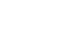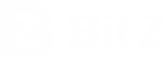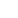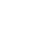English

# 双仓合约概述

BitZ hedging contract is a perpetual contract that allows users to hold two-way positions. A Perpetual Contract is a derivative product that is similar to a traditional Futures Contract, but has a few differing specifications. First of all, perpetual contracts have not expiration date or settlement date. Perpetual swap contract is akin to a margin-based spot market. Therefore, its price is close to the underlying reference Index Price, which is different from futures contract. Futures contract may trade at a significantly different price due to basis. Secondly, the primary mechanism to tether to spot price is Funding.

Currently BitZ provides several digital currency contract transaction including BTC, ETH, EOS, etc. Since contract does not have an expiration date, you don't have to worry about system settlement in the future. It's like owning a bidirectional position in spot market which supports margin trading.

# Mechanics of Hedging Contract Market

When trading perpetual contracts, traders need to know several mechanics of the market. The key components traders need to be aware of are:

1. Fair value Perpetual Swap Contracts are marked according to the Fair Price Marking method. The Mark Price determines unrealized gains and losses and liquidation prices.

2. Initial and Maintenance Margin: the key margin levels determine how much leverage one can trade with and at what price liquidation occurs.

3. Funding: Periodic payments exchanged between the buyer and seller every 8 hours. If the rate is positive, longs will pay and shorts will receive the rate, and vice versa if the rate is negative.-Please note that you will only pay or receive funding when you hold positions at funding timestamp.

4. Funding Timestamps: 00:00 (UTC+8), 08:00 (UTC+8) and 16:00 (UTC+8). Traders can find the current funding rate for contracts on the top right corner of the Trade tab. Similarly you can view this rate in your individual “Contract Specifications”. Historical rates are in the Funding History.

# Funding

Funding fees are not charged by the exchange, but are paid between long and short position.

The purpose of funding fee is to adjust the long and short power through funding cost, which will make the contract price close to the spot index price. BitZ funding rate ranges from -0.375% to 0.375%.

Funding generates every 8 hours at 00:00 (UTC+8), 08:00 (UTC+8) and 16:00 (UTC+8). You will only pay or receive funding if you hold positions at one of these timestamps. If you close your positions prior to the funding timestamp then you will not pay or receive funding.

The funding you pay or receive is calculated as:

Funding = Position Value * Funding Rate

Your position value is irrespective of leverage. For example: I have 10000 USDT, 10 times leverage, and hold a position of 100000 USDT The funding cost is charged or paid by 100000 USDT.

When the Funding Rate is positive, longs pay shorts. When it is negative, shorts pay longs.

# Funding Rate Composition

The Funding Rate is comprised of two main parts: the Interest Rate and the Premium / Discount. This rate aims to keep the transaction price of the perpetual contract in line with spot price. In this way, the hedging contract works like margin-trading spot markets, where buyers and sellers exchange interest payments periodically.

1). Interest rate means using leverage to borrow funds in the platform and all funds have costs.

Taking BTC_USDT hedging contract (USDT settlement) as an example, long is equivalent to borrowing usdt long, short is equivalent to borrowing BTC short, Bitz will fix the interest rate of BTC_USDT perpetual contract (USDT settlement) at 0.03% per day.

2). The premium factor is the premium cost that the contract price needs to pay to the spot index price.

When the contract price is higher than the spot index price for a long time, the funding rate is more than 0, and the long position needs to pay the funding fee to the short position, so that before 3 o'clock, the long position can avoid paying the funding fee, reward the short position to open to gain the funding fee, and make the contract price close to the spot index price.

# Funding Rate Calculations

1). Interest Rate

guide-general-funding-rate-calculations-desc-2

Interest Rate is a function of interest rates between these two currencies:

Interest Rate (I) = (Interest Quote Index - Interest Base Index) / Funding Interval
Where
Interest Base Index = The Interest Rate for borrowing the Base currency
Interest Quote Index = The Interest Rate for borrowing the Quote currency
Funding Interval = 3 (Since funding occurs every 8 hours)

Note: Under each Contract Specification page, the source borrow market is stated for each Interest Index.

For example, in the BTC_USDT contract market, the lending rate of the base currency BTC is 0.03% per day, and the lending rate of the quote currency USDT is 0.06% per day. Due to the large volatility of the lending rate, we set a fixed value recognized by the market.

The hedging contract may trade at a significant premium or discount to the Mark Price. In this situation, a Premium Index will be used to raise or lower the next Funding Rate to match with the contract currently trading.

Each contract's Premium Index is available on the specific instrument's Contract Specifications page and is calculated as follows:

Premium Index (P) = (Max (0, Impact Bid Price - Mark Price) - Max(0, Mark Price - Impact Ask Price)) / Spot Price + Fair Basis used in Mark Price

Note:

The reasonable basis of fair price is the last funding rate.

Weighted depth buying price = the average price of 'margin influence amount' of the buyer in the competition;

Weighted depth selling price = the average price of the 'margin influence amount' of the seller in the competition;

Margin impact refers to the amount that can be traded with margin of 0.1btc (i.e. the ratio of 0.1btc / initial margin), for example, the margin impact of BTC_USD market is 10btc.

# 3). Final Funding Rate Calculation

BitZ calculates the Premium Index (P) and Interest Rate (I) every minute and then performs an 8-Hour Time-Weighted-Average-Price (TWAP) over the series of minute rates.

The Funding Rate is next calculated by the 8-Hour Premium / Discount Component. A +/-0.05% dampener is added.

Funding Rate (F) = Premium Index (P) + clamp (Interest Rate (I) - Premium Index (P), 0.05%, -0.05%)

If: (Interest Rate (I) - Premium Index (P)) is between + / -0.05%, then Funding Rate (F) = P + (I-P) = Interest Rate (I). In other words, the funding rate will be equal to the interest rate.

If: (Interest Rate (I) - Premium Index (P)) > 0.05%, then Funding Rate (F) = Premium Index (P) + 0.05%

If: (Interest Rate (I) - Premium Index (P))< 0.05%, then Funding Rate (F) = Premium Index (P) - 0.05%

# Funding Rate Caps

BitZ imposes caps on the Funding Rate to ensure the maximum leverage can still be utilized. To do this, two caps are imposed:

1) the upper limit of absolute funding rate is (Initial Margin - Maintenance Margin) × 75%.

For example, if the initial margin is 1% and the maintenance margin is 0.5%, then the maximum funding rate = (1% - 0.5%) * 75% = 0.375%.

2) the funding rate shall be within the 'Funding Interval' range and shall not change by more than 75% of the 'Maintenance Margin'.

BitZ does not charge any fees on funding; it is exchanged directly peer-to-peer.